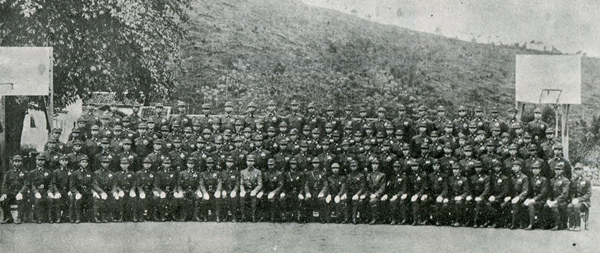•  注 册

# 中央陆军军官学校第十三期第四分校学生总队同学录2021-07-04 20:08:35  来源：静思斋 于岳  点击：说明：本总队毕业于1938年，以下同学均有照片，静思斋可制作此同学录影印本。

如有下列黄埔同学后裔需要先辈照片，请与抗日战争纪念网联系。电话：0731-85531328、15116420702 ; QQ:2652168198;微信号：kz15116420702;邮箱：tougao@krzzjn.com;地址：湖南省长沙市岳麓区英才园1片17栋。

步兵大队第一队：117人

何树德(广东化县) 甄朝杰(广东台山) 黄家兴(广东清远) 杨剑峰(广西藤县)

蔡飞龙(广东揭阳) 罗国宗(广东合浦) 彭福田(广东合浦) 詹志雄(广东惠阳)

杨泽雄(广东揭阳) 区煜华(广东罗定) 谭家騟(广东阳江) 陈彦昌(广东钦县)

林珍儒(广东蕉岭) 李润光(广东顺德) 廖添鑫(广东紫金) 张镳(广东阳江)

黄震(广东合浦) 罗杰仁(广东新丰) 何海瀛(广西崇善) 李泽年(广东信宜)

伍一心(广东恩平) 骆志鹏(广东兴宁) 李朝邦(广东仁化) 张树铮(广东茂名)

钟青(广东五华) 唐将功(四川荣昌) 孔腾(广西宾阳) 陈福光(广东台山)

莫成墉(广东徐闻) 林立军(广东临高) 梁树中(广西容县) 刘向亮(广东茂名)

陈定一(广东兴宁) 黄树光(广东罗定) 敖道高(广东阳江) 范坚青(广东河源)

张兹墉(广东乐昌) 陈宝钦(广东合浦) 劳澄宇(广东化县) 谢剑非(广东东莞)

潘宗干(广东罗定) 叶仕恩(广东惠阳) 罗福初(广西苍梧) 劳耀民(广东阳江)

陈承贻(广东东莞) 罗凯鸣(广东兴宁) 李华权(广西桂平) 黄训经(江西九江)

丁汝嵩(广东东莞) 温若石(广东五华) 雷慎行(广西宾阳) 陈文新(广东梅县)

史立亮(广东琼山) 李礼祥(广西苍梧) 陈伯渊(云南保山) 李宏骑(广东信宜)

陈汉英(广东河源) 骆耀檀(广东从化) 曾福光(广西武鸣) 杨学明(广东惠阳)

唐成金(广东防城) 申炳南(广西平南) 梁介夫(广东大埔) 陈武略(广东陆丰)

范开鉴(广东阳江) 王墨甫(湖南郴县) 梁文威(广东鹤山) 张志鹏(广东兴宁)

张民(广西武宣) 魏天健(广东五华) 黄天才(广西苍梧) 黄铁生(广东番禺)

萧祖藩(广东从化) 杨锦为(广东茂名) 余怀(广东台山) 陈仁政(广东罗定)

陈博济(广东和平) 吴轩(广西武鸣) 刘英贤(广东廉江) 吴辉廷(广西宾阳)

陆友仁(广西宾阳) 招奇超(广东罗定) 王作新(广东东莞) 林参霄(广东茂名)

王道明(广西贵县) 管瑞龙(广东惠阳) 王文萃(广东增城) 傅伟英(广东兴宁)

符国芳(广东文昌) 吴恩铭(广东丰顺) 曾宗铿(广东蕉岭) 陈光中(广东蕉岭)

林琇良(广东合浦) 夏桂枬(广西容县) 邓佩康(广东合浦) 李林(广东茂名)

覃德心(广西武宣) 文国钦(广东惠阳) 全东华(广西昭平) 梁炳桂(广东恩平)

吴鉴(湖南宜章) 许炳彬(广东防城) 温映伟(广东梅县) 钟志达(广东兴宁)

丘子英(广东河源) 陈贵谋(广东化县) 陈以居(广东广宁) 刘享(江西南康)

戴铗(广东惠阳) 赵仲健(广东台山) 何显荣(广东合浦) 潘锦良(广东恩平)

罗志云(广东丰顺) 李谷诒(广东德庆) 杨观光(广东新会) 陈英才(广东琼山)

童伟祺(广东惠阳)

步兵大队第二队：112人

沈步云(广东番禺) 乐声远(广西苍梧) 黄振超(广东兴宁) 张裕恒(广东文昌)

范绍镁(广东合浦) 邓增雄(广东惠阳) 钟英(广东五华) 黄景才(广东台山)

朱兆康(广东增城) 钟锦标(广东东莞) 吴生实(广东琼山) 李孝威(广东信宜)

詹达邦(广东吴川) 何冠球(广东新会) 陈燕模(广西陆川) 谢英(广东龙川)

文毅武(广东惠阳) 张志仁(广东罗定) 吴相英(广西宾阳) 钟万雄(广东五华)

黄治全(广东台山) 彭季彝(广东信宜) 郭仲英(江西吉安) 宋森文(广西平南)

曾子廉(广东揭阳) 林禔(广东信宜) 何国材(广西平南) 何汉珍(广东兴宁)

莫庆云(广东云浮) 杨谭祺(广东惠阳) 张华成(广东五华) 李进昌(广东东莞)

郑兆荣(广东宝安) 陈锐兴(广东德庆) 王国培(贵州独山) 冯邦达(广东阳江)

陈策安(广东琼山) 张宝定(广东东莞) 李光贤(广东灵山) 林璇(广东吴川)

徐尔纯(广东丰顺) 张兆勋(广东信宜) 罗柱(广东东莞) 江燮(广东曲江)

宋文达(广东海丰) 郑叔达(广东番禺) 黄定珠(广东灵山) 彭勋(广东五华)

廖璝珍(广西宾阳) 徐信奇(广东防城) 黄达(广东化县) 邢受章(广东文昌)

陈志仁(广东五华) 王从飞(广东平远) 彭济涉(广东陆丰) 张光洁(广东中山)

张振英(江西大庾) 李汝茂(广东茂名) 何国(广东乐会) 钟介明(广东五华)

杨若愚(广东惠阳) 蔡法(广东遂溪) 胡百濯(广东开平) 谭威(广东罗定)

赖权华(广东灵山) 林松年(广东茂名) 许忠(广东琼山) 邓汉英(广东翁源)

陆雄光(广东新兴) 罗饶华(广东郁南) 马烈通(广东潮阳) 陈守一(广东南海)

廖润才(广东灵山) 余雄欧(广东兴宁) 蔡志良(广东琼山) 何祖炎(广东茂名)

范兆元(广东惠阳) 华秉钺(广东始兴) 练梅清(广东兴宁) 黄楚雄(广东中山)

伍甫宾(广东兴宁) 钟开儒(广东文昌) 伍嘉礼(广东台山) 王三麟(广东琼山)

刘振荣(广东五华) 欧阳鎏(广东三水) 黄应康(广东新会) 何涛(广东始兴)

陈敬诚(朝xian) 周致庸(广东五华) 沈家明(广东罗定) 戴宅卿(广东茂名)

曾育才(广东五华) 胡特奇(广东五华) 魏知新(广东五华) 沈尘智(广西苍梧)

吴刚(广东茂名) 陈其箴(广东兴宁) 苏龙澂(广西玉林) 施达民(广西宾阳)

黄炳辉(江西会昌) 蔡启胜(广东电白) 李zhi强(广东中山) 张满才(广东花县)

林超(广东中山) 周永鑫(广东琼山) 梁起扬(广东阳江) 李育才(广东梅县)

吕德福(广西陆川) 郑道元(广东五华) 覃伯明(广西贵县) 王献梧(广东合浦)

步兵大队第三队：116人

马芬平(广东台山) 官昭洒(广东大埔) 余敏生(广东开平) 霍值(广东南海)

梁剑锋(广西容县) 廖就堂(广东合浦) 赵威(广西博白) 黄应捷(广东惠阳)

李茂燊(广东信宜) 冯文丰(广东合浦) 曾沈秀(广东蕉岭) 陈兆霖(广东顺德)

陈池凤(广东蕉岭) 廖堃(广西宾阳) 黄经魁(广西陆川) 欧阳逑(湖南桂阳)

杨生球(广西宾阳) 秦凤山(湖南桂阳) 陈冠雄(广东罗定) 邓文光(广东防城)

张彭寿(广东茂名) 余镇(广西榴江) 张作民(广东大埔) 周济民(广东琼山)

颜毅民(广东惠阳) 廖有生(广东连县) 温佐周(广东钦县) 李绍祥(广东合浦)

张维(广东茂名) 莫世贤(广东东莞) 苏绍光(广东钦县) 刘懿明(广东和平)

古振中(广西榴江) 蔡嘉言(广东电白) 何龙标(广东开平) 谭增域(广东茂名)

覃竞球(广西容县) 曾奇三(广西武鸣) 莫维畴(广东郁南) 骆国恩(广东博罗)

朱敬华(广东德庆) 张作仁(广东合浦) 黎俊逵(广西钟山) 刘涤中(广东兴宁)

李清泉(广东合浦) 杨剑光(广东化县) 潘超(广东兴宁) 吴捷掎(广西兴业)

廖元斌(广东合浦) 邹维(湖南常宁) 黄宏业(广东惠阳) 颜朗三(广东防城)

李汉泉(广东兴宁) 彭鸿联(广东新兴) 周文光(广西贵县) 覃国斌(广西柳州)

孙一峰(朝xian) 彭志雄(广西玉林) 邓国良(广东惠阳) 刘树毅(广东钦县)

陈善修(广东合浦) 黄文忠(广东鹤山) 段青舞(湖南资兴) 陈有光(广东合浦)

郭李萱(广东合浦) 邹早越(湖南醴陵) 陈均鏐(广东合浦) 黄燊荣(广西苍梧)

许让洪(广东番禺) 梁汝明(广东博罗) 饶启陵(广东梅县) 侯克东(广东梅县)

陈锡安(广东合浦) 李汉初(广东兴宁) 梁杰英(广东开平) 苏元来(广东顺德)

萧振坤(广东兴宁) 阮振荣(广西崇善) 陈文英(广东文昌) 林兴业(广东合浦)

杨焱矗(广东惠阳) 谢福铨(广东郁南) 谢亮基(广东龙川) 梁巨森(广东茂名)

曹强(广东河源) 沈廷芳(广东儋县) 麦增民(广东信宜) 廖雨腾(广东兴宁)

卢茂坤(广东郁南) 范绍(广东惠阳) 谢恳忠(广东乐昌) 罗辅善(广东兴宁)

刘汉达(广东兴宁) 陈东(广西玉林) 李炳华(广东合浦) 翟起(广东钦县)

卞肇琦(四川江津) 赖中强(广西桂平) 陈圣典(广东临高) 梁伟(广东阳江)

廖仲文(湖南郴县) 黄封蕃(广东钦县) 曹才生(广东三水) 丘国祥(广东梅县)

石遂良(广西藤县) 施炽光(广东海丰) 梁昌(广东罗定) 何俊杰(广西平南)

李云鹏(广东兴宁) 梁超汉(广东钦县) 何毅刚(广西玉林) 崔广(广东茂名)

李云廷(广东兴宁) 韦国倩(广西桂平) 陈球(广东五华) 蔡弁英(广东澄海)

步兵大队第四队：115人(1人休学无照片)

张中合(广东合浦) 符逵(广东文昌) 谢文芳(广东罗城?) 苏活林(广东钦县)

陈成东(广东合浦) 李祥(广东龙川) 伍世鸣(广东兴宁) 陈劲锋(广东兴宁)

石国梁(湖南靖县) 丘光(广东梅县) 丘创本(广东潮安) 邓少杰(广东惠阳)

杨士有(广东钦县) 吴岳(广东海康) 李立香(广东兴宁) 刘东初(广东兴宁)

黄觉非(广西昭平) 王云开(广东文昌) 卢天佐(广东德庆) 梁国廉(广东茂名)

何家馨(广西藤县) 吴冠宏(广东兴宁) 黄羲(广西横县) 甘耀驹(广东新兴)

刘玉(湖南桂阳) 陈秀(广西北流) 萧铎天(广东兴宁) 廖绍琼(广西陆川)

邓朝儒(广东云浮) 黄庆增(广东高明) 温耐(广东梅县) 刘莅庄(湖南桂阳)

徐友淇(广东东莞) 王平(广东海康) 何藉光(广东防城) 黄志威(广西贺县)

王凯伦(广东兴宁) 李权(广西陆川) 杨英辉(广东茂名) 陈文英(广东文昌)

杨进鹏(广东惠阳) 黄家琰(广东合浦) 黄步增(广东增城) 刘礼求(广东灵山)

韩子和(广东博罗) 陈仕卿(广东兴宁) 刘在民(广东五华) 李超豪(广东合浦)

何汝钦(广东乐会) 李华(广东平远) 丘锽(广东蕉岭) 苏华(广西容县)

杨雄标(广东五华) 冯彰威(广东钦县) 林子山(广东合浦) 张启锦(广东合浦)

李汉昌(广东化县) 李裕卓(广东阳春) 张国鎏(广东东莞) 林永素(休学)

蒋肃(湖南江华) 辛海强(广东桂平) 陈瑞华(广东惠阳) 程松柏(安徽休宁)

苏寿清(广东罗定) 文维(广东惠阳) 甘伟明(广东防城) 马国豪(广东番禺)

何玉明(广东廉江) 陈国兴(广东肇庆) 韦少泉(广西宾阳) 张衍芬(广西)

沈奇(广西) 王根中(云南云龙) 李宪儒(广东灵山) 甘仁(广西宾阳)

陈清(广东兴宁) 何和民(广东文昌) 黄廷赞(广东台山) 王仲英(广东兴宁)

祁树桂(广东东莞) 梁少忠(广东新兴) 梁汉邦(广东高要) 何清荣(广东兴宁)

古璜() 吴祥勋(四川资阳) 张汉(广东兴宁) 张立中(广东兴宁)

张国球(湖南桂阳) 胡宜生(广东防城) 陈启(广东新会) 彭汉强(广西博白)

宋肇鑫(广东化县) 甘振(广东紫金) 李超葵(广东合浦) 张君良(广西宾阳)

梁国超(广东东莞) 李伟华(广东兴宁) 覃炎兴(广西容县) 陈汉峰(广东新兴)

谢仰文(广东平远) 谢圣理(广东临高) 全科旺(广西玉林) 封尚芳(广西容县)

邱伟雄(广东信宜) 朱威(广东兴宁) 马宽民(广东台山) 李作新(广东东莞)

简耀垣(广东四会) 袁学政(广东合浦) 程升荣(广东茂名) 杨济艰(广东惠阳)

谢文贤(广东陆川) 郑庆林(广东番禺) 许志明(广东琼山)

炮兵队：120人

林英(广东蕉岭) 甘适(广西宁明) 余炳权(广东南海) 黄家齐(广东定安)

陈思谋(广东阳江) 谢参(广西陆川) 蒋健材(广西玉林) 吕镐隆(广西陆川)

黄可敷(广西宾阳) 韦璧臣(广西横县) 秦满林(广东番禺) 廖月桂(广东番禺)

班文海(广西邕宁) 钟善彰(广东合浦) 余永江(广东博罗) 郭卓安(广东合浦)

古国器(广东赤溪) 姚君武(广东增城) 黄明裕(广东惠阳) 李哲诚(广西宾阳)

凌禧德(广东防城) 林桓(广东惠阳) 宁创基(广西玉林) 何清祥(广东兴宁)

黄积发(广东曲江) 李宝光(广东新会) 丘福南(广东惠阳) 胡树中(广东五华)

伍旧斯(云南昭通) 欧阳骧(湖南蓝山) 沈朝谏(广东合浦) 李炳(广东东莞)

陈逵(广东兴宁) 杨熊(广东惠阳) 张士杰(广东兴宁) 廖光华(广东五华)

洪盛茂(广东阳江) 关勋业(广东阳江) 叶璇玑(广东顺德) 张怀(广东五华)

罗汉欧(广东兴宁) 黄其华(广东蕉岭) 罗舜恩(广东惠阳) 何志寅(浙江富阳)

颜建刚(广东连平) 范云麟(广东梅县) 张新锴(广东紫金) 李焕华(广东台山)

李受祺(广东新会) 潘章雄(广东揭阳) 赖春明(广东五华) 吴汉强(广东琼山)

陈政英(广东合浦) 黄柱良(广东东莞) 曾经武(广东兴宁) 陈觐安(广东兴宁)

邬卓宏(广东惠阳) 陈英(广东蕉岭) 陈文生(广西苍梧) 夏维中(贵州平舟)

丘炯(广东梅县) 姚义英(广东平远) 谢增华(广东兴宁) 杨修锵(福建闽侯)

郭朝岳(广东万宁) 廖超寰(广东兴宁) 谢汝清(广东阳江) 陈汉淞(广西陆川)

梁鹤立(广东顺德) 陈开铭(江西南昌) 钟达文(广东蕉岭) 陈达绪(广东阳江)

罗思温(广东惠阳) 伍应皋(广东惠阳) 周楠盛(广东信宜) 谢道佐(广东龙川)

陈永裕(广东高要) 曾鉴超(广东五华) 胡健(湖南永兴) 陈逸(广东揭阳)

黄启礼(广东蕉岭) 程堃(广东茂名) 李翊臣(广东从化) 梁梦麟(广东番禺)

郑勇光(广东琼山) 梁海(广东开平) 蔡如民(广东遂溪) 黄炽昌(广东中山)

刘秉宽(广东梅县) 朱挺英(广东五华) 梁裕宽(广东阳江) 郭启平(广东增城)

饶毓蕃(湖北恩施) 黄之杰(广西陆川) 李超雄(广东信宜) 梁材(广西宾阳)

莫寿松(广东定安) 曹是元(广东阳江) 何天运(广东定安) 万祥群(广东五华)

吴卓儒(广东郁南) 陈传桄(广东信宜) 胡树祺(广东番禺) 冯尧和(广东潮安)

曾一(湖南湘乡) 黄子良(广东蕉岭) 曹任夫(湖南湘潭) 吴家宣(广东海康)

吴冠群(广东五华) 邓根昌(广东蕉岭) 谢远华(广东东莞) 张焕(广东兴宁)

徐杰景(广东东莞) 黄国藻(广东龙川) 曾亚雄(广东化县) 黄秀棻(广西博白)

魏武(广西贵县) 刘冠华(广东五华) 张炳其() 陈懿钦()

工交队工兵区队：62人

谭仲博(广东新会) 曾仲英(江西萍乡) 廖经习(广东丰顺) 曾国梁(广东揭阳)

王禧鸿(广西玉林) 刘禹田(广东中山) 文心存(广东惠阳) 林文兴(广东梅县)

苏清林(广东罗定) 张一谔(广东信宜) 刘逸民(广东惠阳) 陈耀明(广西藤县)

廖永旺(广东兴宁) 邓荦(广东五华) 林兆材(广东番禺) 李维栋(广东新兴)

刘烈(广西博白) 聂匡民(广西苍梧) 王惠光(广东惠阳) 符瑞珍(广东儋县)

陈学贯(广东顺德) 莫若秀(广西怀集) 曹圣修(广东信宜) 谭季英(广东茂名)

张志远(福建宁化) 张龚(广东梅县) 李树安(广东兴宁) 李惠群(广东五华)

向承猛(湖南衡山) 毛治汉(广东和平) 张廷辉(广东惠阳) 黄斌(广东新会)

单庆嵩(广东东莞) 李叶茂(广东东莞) 陆健鸣(广东钦县) 陈侠(广东兴宁)

韩庭辉(广东博罗) 谢静之(广西横县) 云维容(广东文昌) 李如舜(广东德庆)

梁荣昌(广东惠阳) 杨集志(广东惠阳) 方锡圭(广东普宁) 施竹伊(广东新会)

李赐林(广东五华) 钟子纯(广东廉江) 卢国材(广东梅县) 胡峻(江苏上海)

廖志忠(广东兴宁) 叶奇峰(广东惠阳) 刘贤俊(广东台山) 玉美瑄(广东番禺)

郑邦琼(广东琼山) 郭远璠(广东文昌) 罗富基(广东阳江) 林伯继(广东博罗)

陈秉衡(广西蒙山) 林粹(广东茂名) 陈惠(广东罗定) 江流源(广西贺县)

何达先(广东新会) 张荣兴(广东平远)

工交队交通区队：59人

张庆绍(广东五华) 李若栋(广东化县) 周咏辉(广东五华) 张大杰(广东五华)

陈文山(广东合浦) 张启源(广东五华) 周拔恺(广东梅县) 韦文运(广西宾阳)

陈声宏(广东化县) 黄昭德(广东阳春) 李格(广东惠阳) 黄炳申(广东惠阳)

何同方(广西宾阳) 梁介楠(广东中山) 关秉式(广东南海) 陈棨(广东兴宁)

陈善玙(广东合浦) 王辅蕃(广东琼山) 陈耀昌(广东中山) 陈锡康(广东新会)

陈升(广东罗定) 黄启疆(广西桂平) 何胜发(广东兴宁) 罗炯光(广东兴宁)

梅演秀(广东台山) 张略(广西百色) 杨进琪(广东茂名) 王容光(广东灵山)

符大钟(广东文昌) 官家隽(广东始兴) 周那孚(广东化县) 农志雄(广西宁明)

陆雄飞(广西南宁) 颜仲昆(广西百色) 林光杰(广东海丰) 徐崇侃(广东番禺)

卢荣新(广西陆川) 黎锦汉(广东番禺) 黎青山(广西宾阳) 梁导先(广西龙州)

吴祥经(四川资阳) 黄步贤(广东中山) 梁保全(广东茂名) 罗定中(广东博罗)

吴熙城(广东高要) 崔少球(广东东莞) 谢英堃(广西玉林) 周增盛(广东信宜)

张展林(广东罗定) 丘庆勋(广西陆川) 陈鳌(广东海康) 谭秀琨(广东阳江)

潘业传(广东河源) 廖冠洲(广东茂名) 张荣华(广东蕉岭) 王兴亚(广东遂溪)

戴礼钧(广西平南) 吴乾年(广东顺德) 孔昭钵(广东琼东)

黄埔同学录链接(红色字体代表已经上传，可以点击进入)：

中央陆军军官学校南昌分校同学录(六期独立第一大队)(1929年)

中央陆军军官学校第七期武汉分校同学录(1930年)

中央陆军军官学校第十期第一总队同学录(1936年)

中央陆军军官学校第十二期同学录(据1985年该期入伍五十周年纪念册整理)

中央陆军军官学校第十二期广州分校学生总队同学录(1937或1938年)

中央陆军军官学校第十三期同学录(台湾重印本)

中央陆军军官学校第十三期第四分校学生总队同学录(1938年)

中央陆军军官学校第十四期第一总队同学录( 1938年)

中央陆军军官学校第十四期第二总队同学录(据1987年该总队入伍五十周年纪念册整理)

中央陆军军官学校第十五期一总队同学录

中央陆军军官学校第十六期第二总队同学录(成都本校)(1939年)

中央陆军军官学校第十六期第九总队同学录(第四分校)(1939年)

中央陆军军官学校第十六期第十二总队同学录(第六分校)

中央陆军军官学校第十六期第十四总队同学录( 第七分校)(1941年)

中央陆军军官学校第十六期第十五总队同学录(第七分校)(1941年)

中央陆军军官学校第五分校第十六期学生第十八总队同学通讯录(原第-集团军干部总队)(1940年)

中央陆军军官学校第十七期学生第五总队同学录( 第四分校)(1941年)

中央陆军军官学校第十七期学生第十七总队同学录(第六分校)(1941年)

中央陆军军官学校第二分校第十七期第二十一总队官佐学员生通讯录(1941年)

中央陆军军官学校第十七期二十六总队华侨生毕业同学录( 第四分校)(1941年)

中央陆军军官学校第十八期学生第一总队同学录 (成都本校)(1943年)

中央陆军军官学校第十八期第十-总队同学录(鲁干班)

中央陆军军官学校第十九期学生第一总队同学录 ( 成都本校)(1945年)

中央陆军军官学校第十九期学生第八总队第一大队毕业同学录(第四分校)(1944年)

中央陆军军官学校十九期十三总队同学录( 第七分校)(1946年)

中央陆军军官学校十九期十六总队同学录(鲁干班，内含18期11总队名录) (1944年)

陆军军官学校第十九期炮科学生第四大队通讯录(陆军炮兵学校代训)(1946年)

陆军军官学校第二十一期同学录( 西安督训处部分)(1947年)

陆军军官学校第二十二期第一总队同学录(1949年)

陆军军官学校第二十二期第二总队同学录(1949年)

陆军军官学校第二十二期第三总队同学录(1949年)

陆军军官学校第二十三期毕业同学录

中央陆军军官学校政训研究班第一-期同学录

中央陆军军官学校高等教育班第七期同学录(1941年)

陆军军官学校军官高等教育班第十-期同学录(1946年)

中央陆军军官学校军官训练班第三期同学录(洛阳分校，残本不全)(1935年)

陆军军官学校第二军官训练班第十五期毕业同学录

陆军军官学校第二十五期同学录(台湾省黄埔)(1954年)

2、民国其他军事学校系列:

中央航空学校同学录(1-6期) (约1936年)

空军军官学校第十五期同学录(1974年编印)

空军军官学校二十六期毕业同学录(1948年)

广东航空学校第五期同学录(1932年)

空军通信学校第十期正科班同学通信录(1950年)

留学日本陆军步兵学校第十期同学录(约1931年)

陆军大学第六期同学录(1922年)

陆军大学特别班第五期同学录(1941年)

云南讲武堂第21期同学录(讨逆军)(1931年_

陆军步兵学校第四期同学录(西南分校)(1943年)

陆军步兵学校教官训练班同学录(1947年)

陆军步兵学校高级班第一期同学录(1949年)

陆军机械化学校战车/技术学生第三期毕业纪念特刊( 黄埔17期战车兵科)(约1944年)

陆军机械化学校学生第七期毕业同学录(学籍叙黄埔21期战车兵科)(1947年)

军事委员会战时工作干部训练团第一-团第一 期学生 第一总队毕业同学通讯录(1939年)中文域名：www.抗日战争纪念网.com　主办单位：长沙市抗战文化研究会　技术支持：刘庆为